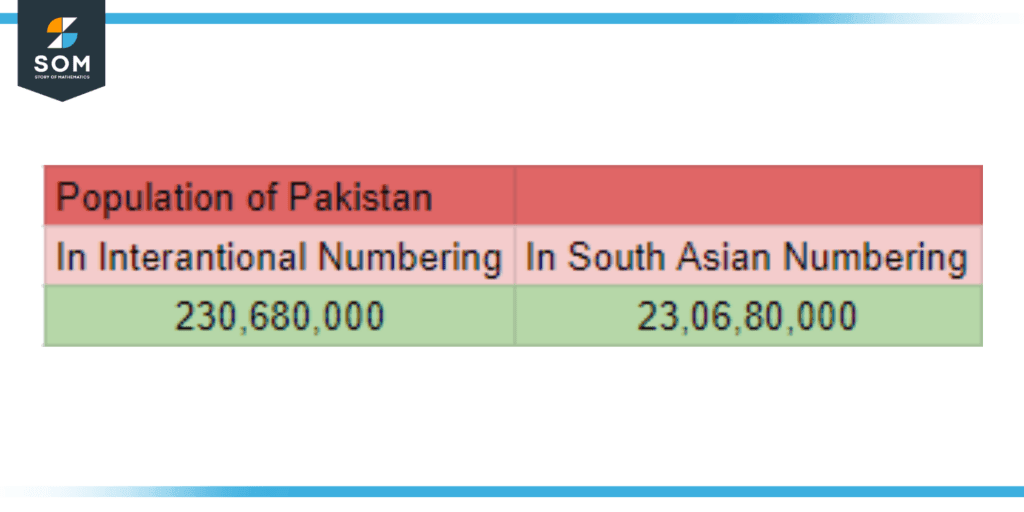# Crore|Definition & Meaning

## Definition

In the South Asian numbering system, crore is a value equivalent to ten million value in the International numbering system. It can also be denoted in the form of 10$^7$ in scientific notation. It is written as 1,00,00,000 in the South Asian numbering system, which is denoted as 10,000,000 in the international numbering system.Figure 1: Population of Pakistan written in crore numbering.

Unlike the three-digit separator technique in the international numbering system, the South Asian countries adopt a 2-2-3 separation method hence the crore is having 7 zeros with 3 then 2 and 2 digit separators. This numbering system is usually implemented in South Asian Countries that include Pakistan, India, Bangladesh, Nepal, Myanmar, Srilanka, Bhutan, and Afghanistan.

## The South Asian Numbering System

The South Asian Numbering System is a numbering system that is adopted by South Asian countries that include Pakistan, India, Bangladesh, Nepal, Myanmar, Srilanka, Bhutan, and Afghanistan, to express large numbers. It is unique in the usage of 2 digit separator after the thousand value instead of the 3-digit separators used in the international numbering system.

Furthermore, this numbering system uses the words “lakh” “crore” “arab” and “kharab” to denote large sums of numbers. This numbering scheme has been used since the Vedic Age (c. 1500 – c. 500 BCE). These have been a convention for the local people and have continued to use since then.

The term “lakh” is used to denote values that in the International numbering system would be equal to one hundred thousand. A hundred thousand number is denoted as “100,000” whereas, in South Asian numbering, lakh is denoted as “1,00,000.” We can observe the use of digit separators grouped for 2 numbers after the initial three-number grouping.

Moreover, the term “crore” has been used to denote values equal to ten million in the international numbering system. It is represented as 1,00,00,000 in the South Asian system as compared to the ten million, 10,000,000. It is a very easy-to-use convention used in the Indian subcontinent.Figure 2: Comparison between the International and the South Asian numbering systems.

### History of the South Asian Numbering System

South Asian numbering system dates back to the early Vedic Age, where this was a common usage of defining large values. They have been since using this numbering system and is widely accepted in the Indian Subcontinent over the usage of the international numbering system. Sri Lanka has adopted the International numbering system but the rest of the Indian subcontinent still abides by its conventions and culture.

Moreover, the terms Lakh and Crore are derived from the Sanskrit names “Laksha” and “koti” respectively. They then mixed with other variants over time such as “krodi” in the Prankit language to become the words that we listen to in the current time.

Furthermore, there are more names with higher values than crore given in the South Asian Numbering system is different from the usual International numbering system. The figure below shows the values greater than a crore and has their names with the scientific value in the standard form beside it.Figure 3: The higher values in the South Asian numbering system with their respective scientific counterparts

## Examples Depicting the Usage of Crore and South Asian Numbering System

Below are a few examples showing how the south Asian numbering system is utilized in calculations and representing answers that contain large values.

### Example 1

A lump of coal has a heating value of 30000kJ/kg for a 600 kW coal-fired power plant. If the efficiency of the plant is 25%, find the total amount of coal supplied to the power plant in one hour and calculate the cost of the coal considering the cost of coal is Rs 200/kg.

### Solution

In this problem, we are given a heating value of 30000 kJ/kg for a 600 kW power plant. First, we need to find the total input power of the power plant by dividing the efficiency by the rated value of the power plant:

Input power of the power plant  = $\dfrac{600}{0.25}$

Input power of the power plant = 2000 MW

Input power of the power plant = 2000 x 10$\mathsf{^3}$ kW

Now, we need to find the amount of coal used to run this power plant per second.

Coal Required (in kg/sec) = $\dfrac{2000 \times 10^3}{30000}$

Coal Required = 66.67 kg/s

Now, the coal required for one hour of running the power plant will be equal to:

Total Coal Required = 66.67 x 3600

Total Coal Required = 240000 kg

So, we require 240000 kg of coal to run this power plant for one hour. Let us calculate the total cost it takes to buy this amount of coal.

Cost of Coal = 240000 x 200

Cost of Coal = Rs. 48000000

Hence, the total cost of coal is Rs. 4,80,00,000, which can be said as 4 crore 80 lakh rupees worth of coal was used to run a coal-fired power plant for one hour.

### Example 2

A salesman buys a large mansion for Rs. 15 crore (Rs. 15,00,00,000) and lives in it for 2 years. After that, he sells the mansion for Rs 13 crore and 50 lakh (Rs. 13,50,00,000). The annual tax he paid was at the rate of 3% of the cost of the mansion. Find the loss amount that the salesman faced.

### Solution

The tax incurred to the salesman on buying the Rs. 15,00,00,000 mansion is calculated as:

Tax of mansion for 2 years = 150000000 x 0.03

Tax of mansion for 2 years = Rs. 4500000

Hence, the tax is Rs. 45,00,000 or Rs. 45 Lakh.

The total loss of the salesman over two years can be calculated as:

Total Loss = 150000000 – 4500000 – 135000000

Total Loss = Rs. 10500000

Thus, the total loss of the salesman is Rs. 1,05,00,000 or Rs. 1 crore and 5 lakh.